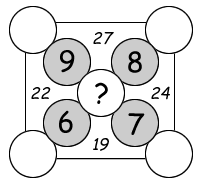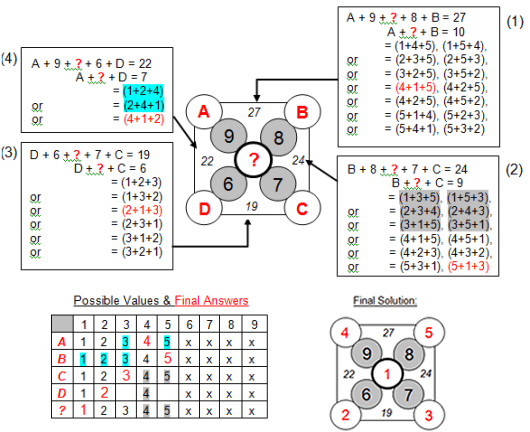Select Page

The four numbers in italics outside the nine circles represent the sum of the numbers in the five surrounding circles.  The numbers in the circles can only be 1 to 9 and each number can be used only once.  Four numbers have been provided to get you started.  Find the number that should be in the center of the X.### Solution### Solution explanationComments on solving the puzzle; a process of eliminating possible values as answers:

Values 6, 7, 8 & 9 are provided and can not be reused.

Starting at Equation (1), unknowns A, B & ? can only be equal to 1, 2, 3, 4 or 5 as shown. All other combinations can not include either a 6, 7, 8 or 9 value.

Equation (2): unknowns B, C & ? can also be equal to 1, 2, 3, 4 or 5 as shown.

Equation (3): unknowns C, D & ? can only be equal to 1, 2 or 3 as shown. Comparing with Equation (2), values 4 & 5 can be eliminated as possible values for C & ?. As a result, unknown B can still be equal to 4 or 5 as shown.

Equation (4): unknowns D, A & ? can be equal to 1, 2 or 4 as shown. Comparing with Equation (3), value 4 can be eliminated as a possible value for D & ?. As a result, the final answer for A can only be equal to 4, ? equal to 1 & D equal to 2 as shown. Comparing with notes (1) & (2), the final answers for B can only be equal to 5 and C equal to 3.

Now insert your final answers into the original puzzle and verify the resulting sums.

## Feedback

There are more than one way of doing these puzzles and may well be more than one answer.  Please let me and others know what alternatives you find by commenting below.  We also welcome general comments on the subject and any feedback you’d like to give.   If you have a question that need a response from me or you would like to contact me privately, please use the contact form.

## Get more puzzles!

If you’ve enjoyed doing the puzzles, consider ordering the book; 150+ of the best puzzles in a handy pocket sized format. Click here for full details.

### Share This

Share this post with your friends!